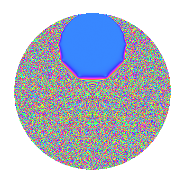Properties

 Label 3.44.a.aLevel 3 Weight 44 Character orbit 3.a Self dual Yes Analytic conductor 35.133 Analytic rank 1 Dimension 3 CM No Inner twists 1

Related objects

Newspace parameters

 Level: $$N$$ = $$3$$ Weight: $$k$$ = $$44$$ Character orbit: $$[\chi]$$ = 3.a (trivial)

Newform invariants

 Self dual: Yes Analytic conductor: $$35.1331186037$$ Analytic rank: $$1$$ Dimension: $$3$$ Coefficient field: $$\mathbb{Q}[x]/(x^{3} - \cdots)$$ Coefficient ring: $$\Z[a_1, \ldots, a_{5}]$$ Coefficient ring index: $$2^{13}\cdot 3^{5}\cdot 5\cdot 11$$ Fricke sign: $$-1$$ Sato-Tate group: $\mathrm{SU}(2)$

$q$-expansion

Coefficients of the $$q$$-expansion are expressed in terms of a basis $$1,\beta_1,\beta_2$$ for the coefficient ring described below. We also show the integral $$q$$-expansion of the trace form.

 $$f(q)$$ $$=$$ $$q$$ $$+ ( 1619008 - \beta_{1} ) q^{2}$$ $$+ 10460353203 q^{3}$$ $$+ ( -593686009856 - 3392488 \beta_{1} + 4 \beta_{2} ) q^{4}$$ $$+ ( -169251107035090 + 87483970 \beta_{1} - 1317 \beta_{2} ) q^{5}$$ $$+ ( 16935395518482624 - 10460353203 \beta_{1} ) q^{6}$$ $$+ ( -544389767902363096 + 407863957886 \beta_{1} + 1184197 \beta_{2} ) q^{7}$$ $$+ ( 3732086898157617152 + 606520958464 \beta_{1} + 19428096 \beta_{2} ) q^{8}$$ $$+ 109418989131512359209 q^{9}$$ $$+O(q^{10})$$ $$q$$ $$+(1619008 - \beta_{1}) q^{2}$$ $$+10460353203 q^{3}$$ $$+(-593686009856 - 3392488 \beta_{1} + 4 \beta_{2}) q^{4}$$ $$+(-169251107035090 + 87483970 \beta_{1} - 1317 \beta_{2}) q^{5}$$ $$+(16935395518482624 - 10460353203 \beta_{1}) q^{6}$$ $$+(-544389767902363096 + 407863957886 \beta_{1} + 1184197 \beta_{2}) q^{7}$$ $$+(3732086898157617152 + 606520958464 \beta_{1} + 19428096 \beta_{2}) q^{8}$$ $$+$$$$10\!\cdots\!09$$$$q^{9}$$ $$+(-$$$$76\!\cdots\!40$$$$+ 1235354107077970 \beta_{1} - 2278729792 \beta_{2}) q^{10}$$ $$+(-$$$$92\!\cdots\!40$$$$+ 7617306526252796 \beta_{1} - 14933373558 \beta_{2}) q^{11}$$ $$+(-$$$$62\!\cdots\!68$$$$- 35486622716939064 \beta_{1} + 41841412812 \beta_{2}) q^{12}$$ $$+(-$$$$33\!\cdots\!50$$$$- 202202396925505444 \beta_{1} + 641923722778 \beta_{2}) q^{13}$$ $$+(-$$$$31\!\cdots\!76$$$$+ 448634534786039896 \beta_{1} + 102843306048 \beta_{2}) q^{14}$$ $$+(-$$$$17\!\cdots\!70$$$$+ 915113225800655910 \beta_{1} - 13776285168351 \beta_{2}) q^{15}$$ $$+($$$$78\!\cdots\!12$$$$+ 13746042266337042432 \beta_{1} - 9157309919232 \beta_{2}) q^{16}$$ $$+(-$$$$53\!\cdots\!94$$$$+ 90683027194690706820 \beta_{1} + 201871787631414 \beta_{2}) q^{17}$$ $$+($$$$17\!\cdots\!72$$$$-$$$$10\!\cdots\!09$$$$\beta_{1}) q^{18}$$ $$+(-$$$$10\!\cdots\!88$$$$+$$$$40\!\cdots\!04$$$$\beta_{1} - 2147995640621886 \beta_{2}) q^{19}$$ $$+(-$$$$66\!\cdots\!20$$$$+$$$$37\!\cdots\!60$$$$\beta_{1} + 3305756267279544 \beta_{2}) q^{20}$$ $$+(-$$$$56\!\cdots\!88$$$$+$$$$42\!\cdots\!58$$$$\beta_{1} + 12387118881932991 \beta_{2}) q^{21}$$ $$+(-$$$$57\!\cdots\!08$$$$+$$$$33\!\cdots\!40$$$$\beta_{1} - 52339689282150272 \beta_{2}) q^{22}$$ $$+($$$$38\!\cdots\!68$$$$-$$$$60\!\cdots\!28$$$$\beta_{1} + 32453105180134026 \beta_{2}) q^{23}$$ $$+($$$$39\!\cdots\!56$$$$+$$$$63\!\cdots\!92$$$$\beta_{1} + 203224746221791488 \beta_{2}) q^{24}$$ $$+($$$$60\!\cdots\!75$$$$-$$$$14\!\cdots\!00$$$$\beta_{1} - 758897906364611660 \beta_{2}) q^{25}$$ $$+($$$$59\!\cdots\!12$$$$-$$$$47\!\cdots\!90$$$$\beta_{1} + 1748929988964422784 \beta_{2}) q^{26}$$ $$+$$$$11\!\cdots\!27$$$$q^{27}$$ $$+(-$$$$28\!\cdots\!48$$$$+$$$$29\!\cdots\!48$$$$\beta_{1} - 12060227383697492832 \beta_{2}) q^{28}$$ $$+(-$$$$94\!\cdots\!86$$$$-$$$$12\!\cdots\!42$$$$\beta_{1} + 20892595961060032401 \beta_{2}) q^{29}$$ $$+(-$$$$79\!\cdots\!20$$$$+$$$$12\!\cdots\!10$$$$\beta_{1} - 23836318478518723776 \beta_{2}) q^{30}$$ $$+(-$$$$18\!\cdots\!12$$$$+$$$$35\!\cdots\!42$$$$\beta_{1} +$$$$13\!\cdots\!53$$$$\beta_{2}) q^{31}$$ $$+(-$$$$96\!\cdots\!96$$$$+$$$$17\!\cdots\!16$$$$\beta_{1} -$$$$23\!\cdots\!48$$$$\beta_{2}) q^{32}$$ $$+(-$$$$96\!\cdots\!20$$$$+$$$$79\!\cdots\!88$$$$\beta_{1} -$$$$15\!\cdots\!74$$$$\beta_{2}) q^{33}$$ $$+(-$$$$59\!\cdots\!92$$$$+$$$$74\!\cdots\!34$$$$\beta_{1} - 67083608408202293376 \beta_{2}) q^{34}$$ $$+(-$$$$12\!\cdots\!40$$$$-$$$$46\!\cdots\!80$$$$\beta_{1} +$$$$16\!\cdots\!58$$$$\beta_{2}) q^{35}$$ $$+(-$$$$64\!\cdots\!04$$$$-$$$$37\!\cdots\!92$$$$\beta_{1} +$$$$43\!\cdots\!36$$$$\beta_{2}) q^{36}$$ $$+(-$$$$25\!\cdots\!26$$$$-$$$$15\!\cdots\!64$$$$\beta_{1} -$$$$37\!\cdots\!36$$$$\beta_{2}) q^{37}$$ $$+(-$$$$39\!\cdots\!36$$$$+$$$$32\!\cdots\!48$$$$\beta_{1} -$$$$47\!\cdots\!12$$$$\beta_{2}) q^{38}$$ $$+(-$$$$34\!\cdots\!50$$$$-$$$$21\!\cdots\!32$$$$\beta_{1} +$$$$67\!\cdots\!34$$$$\beta_{2}) q^{39}$$ $$+(-$$$$25\!\cdots\!00$$$$+$$$$15\!\cdots\!00$$$$\beta_{1} +$$$$98\!\cdots\!80$$$$\beta_{2}) q^{40}$$ $$+(-$$$$50\!\cdots\!22$$$$-$$$$21\!\cdots\!68$$$$\beta_{1} -$$$$13\!\cdots\!78$$$$\beta_{2}) q^{41}$$ $$+(-$$$$33\!\cdots\!28$$$$+$$$$46\!\cdots\!88$$$$\beta_{1} +$$$$10\!\cdots\!44$$$$\beta_{2}) q^{42}$$ $$+(-$$$$16\!\cdots\!96$$$$+$$$$14\!\cdots\!44$$$$\beta_{1} +$$$$35\!\cdots\!70$$$$\beta_{2}) q^{43}$$ $$+(-$$$$19\!\cdots\!24$$$$+$$$$85\!\cdots\!20$$$$\beta_{1} -$$$$77\!\cdots\!88$$$$\beta_{2}) q^{44}$$ $$+(-$$$$18\!\cdots\!10$$$$+$$$$95\!\cdots\!30$$$$\beta_{1} -$$$$14\!\cdots\!53$$$$\beta_{2}) q^{45}$$ $$+($$$$40\!\cdots\!88$$$$-$$$$16\!\cdots\!48$$$$\beta_{1} +$$$$29\!\cdots\!48$$$$\beta_{2}) q^{46}$$ $$+($$$$17\!\cdots\!12$$$$-$$$$41\!\cdots\!68$$$$\beta_{1} +$$$$50\!\cdots\!22$$$$\beta_{2}) q^{47}$$ $$+($$$$82\!\cdots\!36$$$$+$$$$14\!\cdots\!96$$$$\beta_{1} -$$$$95\!\cdots\!96$$$$\beta_{2}) q^{48}$$ $$+($$$$39\!\cdots\!01$$$$+$$$$23\!\cdots\!36$$$$\beta_{1} -$$$$14\!\cdots\!52$$$$\beta_{2}) q^{49}$$ $$+($$$$17\!\cdots\!00$$$$-$$$$33\!\cdots\!75$$$$\beta_{1} -$$$$53\!\cdots\!60$$$$\beta_{2}) q^{50}$$ $$+(-$$$$55\!\cdots\!82$$$$+$$$$94\!\cdots\!60$$$$\beta_{1} +$$$$21\!\cdots\!42$$$$\beta_{2}) q^{51}$$ $$+($$$$65\!\cdots\!36$$$$-$$$$85\!\cdots\!20$$$$\beta_{1} -$$$$12\!\cdots\!40$$$$\beta_{2}) q^{52}$$ $$+($$$$10\!\cdots\!78$$$$+$$$$57\!\cdots\!02$$$$\beta_{1} +$$$$38\!\cdots\!57$$$$\beta_{2}) q^{53}$$ $$+($$$$18\!\cdots\!16$$$$-$$$$11\!\cdots\!27$$$$\beta_{1}) q^{54}$$ $$+($$$$24\!\cdots\!20$$$$-$$$$95\!\cdots\!60$$$$\beta_{1} +$$$$20\!\cdots\!16$$$$\beta_{2}) q^{55}$$ $$+($$$$21\!\cdots\!40$$$$+$$$$77\!\cdots\!00$$$$\beta_{1} -$$$$19\!\cdots\!28$$$$\beta_{2}) q^{56}$$ $$+(-$$$$11\!\cdots\!64$$$$+$$$$42\!\cdots\!12$$$$\beta_{1} -$$$$22\!\cdots\!58$$$$\beta_{2}) q^{57}$$ $$+($$$$55\!\cdots\!88$$$$-$$$$27\!\cdots\!14$$$$\beta_{1} +$$$$81\!\cdots\!04$$$$\beta_{2}) q^{58}$$ $$+(-$$$$59\!\cdots\!92$$$$+$$$$17\!\cdots\!36$$$$\beta_{1} -$$$$22\!\cdots\!36$$$$\beta_{2}) q^{59}$$ $$+(-$$$$69\!\cdots\!60$$$$+$$$$39\!\cdots\!80$$$$\beta_{1} +$$$$34\!\cdots\!32$$$$\beta_{2}) q^{60}$$ $$+(-$$$$41\!\cdots\!06$$$$-$$$$87\!\cdots\!96$$$$\beta_{1} -$$$$17\!\cdots\!68$$$$\beta_{2}) q^{61}$$ $$+(-$$$$22\!\cdots\!52$$$$-$$$$14\!\cdots\!48$$$$\beta_{1} +$$$$62\!\cdots\!40$$$$\beta_{2}) q^{62}$$ $$+(-$$$$59\!\cdots\!64$$$$+$$$$44\!\cdots\!74$$$$\beta_{1} +$$$$12\!\cdots\!73$$$$\beta_{2}) q^{63}$$ $$+(-$$$$32\!\cdots\!12$$$$+$$$$17\!\cdots\!40$$$$\beta_{1} -$$$$33\!\cdots\!36$$$$\beta_{2}) q^{64}$$ $$+(-$$$$85\!\cdots\!80$$$$+$$$$20\!\cdots\!40$$$$\beta_{1} +$$$$89\!\cdots\!26$$$$\beta_{2}) q^{65}$$ $$+(-$$$$60\!\cdots\!24$$$$+$$$$34\!\cdots\!20$$$$\beta_{1} -$$$$54\!\cdots\!16$$$$\beta_{2}) q^{66}$$ $$+($$$$43\!\cdots\!16$$$$-$$$$72\!\cdots\!40$$$$\beta_{1} +$$$$22\!\cdots\!08$$$$\beta_{2}) q^{67}$$ $$+(-$$$$90\!\cdots\!56$$$$-$$$$26\!\cdots\!08$$$$\beta_{1} -$$$$21\!\cdots\!84$$$$\beta_{2}) q^{68}$$ $$+($$$$40\!\cdots\!04$$$$-$$$$63\!\cdots\!84$$$$\beta_{1} +$$$$33\!\cdots\!78$$$$\beta_{2}) q^{69}$$ $$+($$$$62\!\cdots\!60$$$$-$$$$76\!\cdots\!80$$$$\beta_{1} +$$$$43\!\cdots\!08$$$$\beta_{2}) q^{70}$$ $$+($$$$74\!\cdots\!72$$$$+$$$$47\!\cdots\!60$$$$\beta_{1} -$$$$13\!\cdots\!02$$$$\beta_{2}) q^{71}$$ $$+($$$$40\!\cdots\!68$$$$+$$$$66\!\cdots\!76$$$$\beta_{1} +$$$$21\!\cdots\!64$$$$\beta_{2}) q^{72}$$ $$+($$$$10\!\cdots\!18$$$$+$$$$89\!\cdots\!92$$$$\beta_{1} -$$$$57\!\cdots\!40$$$$\beta_{2}) q^{73}$$ $$+($$$$42\!\cdots\!44$$$$+$$$$25\!\cdots\!46$$$$\beta_{1} +$$$$52\!\cdots\!60$$$$\beta_{2}) q^{74}$$ $$+($$$$63\!\cdots\!25$$$$-$$$$15\!\cdots\!00$$$$\beta_{1} -$$$$79\!\cdots\!80$$$$\beta_{2}) q^{75}$$ $$+(-$$$$15\!\cdots\!68$$$$+$$$$95\!\cdots\!24$$$$\beta_{1} -$$$$11\!\cdots\!36$$$$\beta_{2}) q^{76}$$ $$+($$$$53\!\cdots\!48$$$$-$$$$68\!\cdots\!28$$$$\beta_{1} +$$$$22\!\cdots\!80$$$$\beta_{2}) q^{77}$$ $$+($$$$61\!\cdots\!36$$$$-$$$$49\!\cdots\!70$$$$\beta_{1} +$$$$18\!\cdots\!52$$$$\beta_{2}) q^{78}$$ $$+(-$$$$14\!\cdots\!60$$$$+$$$$10\!\cdots\!50$$$$\beta_{1} -$$$$24\!\cdots\!83$$$$\beta_{2}) q^{79}$$ $$+($$$$17\!\cdots\!60$$$$-$$$$14\!\cdots\!80$$$$\beta_{1} -$$$$15\!\cdots\!72$$$$\beta_{2}) q^{80}$$ $$+$$$$11\!\cdots\!81$$$$q^{81}$$ $$+(-$$$$69\!\cdots\!32$$$$+$$$$47\!\cdots\!02$$$$\beta_{1} +$$$$66\!\cdots\!64$$$$\beta_{2}) q^{82}$$ $$+($$$$29\!\cdots\!56$$$$-$$$$90\!\cdots\!92$$$$\beta_{1} -$$$$21\!\cdots\!90$$$$\beta_{2}) q^{83}$$ $$+(-$$$$29\!\cdots\!44$$$$+$$$$30\!\cdots\!44$$$$\beta_{1} -$$$$12\!\cdots\!96$$$$\beta_{2}) q^{84}$$ $$+(-$$$$20\!\cdots\!40$$$$-$$$$98\!\cdots\!80$$$$\beta_{1} +$$$$23\!\cdots\!38$$$$\beta_{2}) q^{85}$$ $$+(-$$$$35\!\cdots\!40$$$$+$$$$19\!\cdots\!16$$$$\beta_{1} -$$$$51\!\cdots\!56$$$$\beta_{2}) q^{86}$$ $$+(-$$$$98\!\cdots\!58$$$$-$$$$13\!\cdots\!26$$$$\beta_{1} +$$$$21\!\cdots\!03$$$$\beta_{2}) q^{87}$$ $$+(-$$$$28\!\cdots\!28$$$$+$$$$11\!\cdots\!24$$$$\beta_{1} +$$$$51\!\cdots\!28$$$$\beta_{2}) q^{88}$$ $$+($$$$21\!\cdots\!02$$$$+$$$$24\!\cdots\!04$$$$\beta_{1} -$$$$14\!\cdots\!20$$$$\beta_{2}) q^{89}$$ $$+(-$$$$83\!\cdots\!60$$$$+$$$$13\!\cdots\!30$$$$\beta_{1} -$$$$24\!\cdots\!28$$$$\beta_{2}) q^{90}$$ $$+($$$$45\!\cdots\!08$$$$+$$$$23\!\cdots\!72$$$$\beta_{1} -$$$$15\!\cdots\!34$$$$\beta_{2}) q^{91}$$ $$+($$$$12\!\cdots\!24$$$$-$$$$36\!\cdots\!24$$$$\beta_{1} +$$$$81\!\cdots\!12$$$$\beta_{2}) q^{92}$$ $$+(-$$$$19\!\cdots\!36$$$$+$$$$37\!\cdots\!26$$$$\beta_{1} +$$$$14\!\cdots\!59$$$$\beta_{2}) q^{93}$$ $$+($$$$26\!\cdots\!40$$$$-$$$$12\!\cdots\!32$$$$\beta_{1} +$$$$24\!\cdots\!64$$$$\beta_{2}) q^{94}$$ $$+($$$$31\!\cdots\!00$$$$-$$$$59\!\cdots\!00$$$$\beta_{1} -$$$$14\!\cdots\!60$$$$\beta_{2}) q^{95}$$ $$+(-$$$$10\!\cdots\!88$$$$+$$$$18\!\cdots\!48$$$$\beta_{1} -$$$$25\!\cdots\!44$$$$\beta_{2}) q^{96}$$ $$+($$$$20\!\cdots\!26$$$$+$$$$21\!\cdots\!80$$$$\beta_{1} -$$$$24\!\cdots\!92$$$$\beta_{2}) q^{97}$$ $$+(-$$$$67\!\cdots\!20$$$$+$$$$10\!\cdots\!59$$$$\beta_{1} -$$$$30\!\cdots\!16$$$$\beta_{2}) q^{98}$$ $$+(-$$$$10\!\cdots\!60$$$$+$$$$83\!\cdots\!64$$$$\beta_{1} -$$$$16\!\cdots\!22$$$$\beta_{2}) q^{99}$$ $$+O(q^{100})$$ $$\operatorname{Tr}(f)(q)$$ $$=$$ $$3q$$ $$\mathstrut +\mathstrut 4857024q^{2}$$ $$\mathstrut +\mathstrut 31381059609q^{3}$$ $$\mathstrut -\mathstrut 1781058029568q^{4}$$ $$\mathstrut -\mathstrut 507753321105270q^{5}$$ $$\mathstrut +\mathstrut 50806186555447872q^{6}$$ $$\mathstrut -\mathstrut 1633169303707089288q^{7}$$ $$\mathstrut +\mathstrut 11196260694472851456q^{8}$$ $$\mathstrut +\mathstrut 328256967394537077627q^{9}$$ $$\mathstrut +\mathstrut O(q^{10})$$ $$3q$$ $$\mathstrut +\mathstrut 4857024q^{2}$$ $$\mathstrut +\mathstrut 31381059609q^{3}$$ $$\mathstrut -\mathstrut 1781058029568q^{4}$$ $$\mathstrut -\mathstrut 507753321105270q^{5}$$ $$\mathstrut +\mathstrut 50806186555447872q^{6}$$ $$\mathstrut -\mathstrut 1633169303707089288q^{7}$$ $$\mathstrut +\mathstrut 11196260694472851456q^{8}$$ $$\mathstrut +\mathstrut$$$$32\!\cdots\!27$$$$q^{9}$$ $$\mathstrut -\mathstrut$$$$22\!\cdots\!20$$$$q^{10}$$ $$\mathstrut -\mathstrut$$$$27\!\cdots\!20$$$$q^{11}$$ $$\mathstrut -\mathstrut$$$$18\!\cdots\!04$$$$q^{12}$$ $$\mathstrut -\mathstrut$$$$99\!\cdots\!50$$$$q^{13}$$ $$\mathstrut -\mathstrut$$$$94\!\cdots\!28$$$$q^{14}$$ $$\mathstrut -\mathstrut$$$$53\!\cdots\!10$$$$q^{15}$$ $$\mathstrut +\mathstrut$$$$23\!\cdots\!36$$$$q^{16}$$ $$\mathstrut -\mathstrut$$$$16\!\cdots\!82$$$$q^{17}$$ $$\mathstrut +\mathstrut$$$$53\!\cdots\!16$$$$q^{18}$$ $$\mathstrut -\mathstrut$$$$32\!\cdots\!64$$$$q^{19}$$ $$\mathstrut -\mathstrut$$$$19\!\cdots\!60$$$$q^{20}$$ $$\mathstrut -\mathstrut$$$$17\!\cdots\!64$$$$q^{21}$$ $$\mathstrut -\mathstrut$$$$17\!\cdots\!24$$$$q^{22}$$ $$\mathstrut +\mathstrut$$$$11\!\cdots\!04$$$$q^{23}$$ $$\mathstrut +\mathstrut$$$$11\!\cdots\!68$$$$q^{24}$$ $$\mathstrut +\mathstrut$$$$18\!\cdots\!25$$$$q^{25}$$ $$\mathstrut +\mathstrut$$$$17\!\cdots\!36$$$$q^{26}$$ $$\mathstrut +\mathstrut$$$$34\!\cdots\!81$$$$q^{27}$$ $$\mathstrut -\mathstrut$$$$84\!\cdots\!44$$$$q^{28}$$ $$\mathstrut -\mathstrut$$$$28\!\cdots\!58$$$$q^{29}$$ $$\mathstrut -\mathstrut$$$$23\!\cdots\!60$$$$q^{30}$$ $$\mathstrut -\mathstrut$$$$56\!\cdots\!36$$$$q^{31}$$ $$\mathstrut -\mathstrut$$$$29\!\cdots\!88$$$$q^{32}$$ $$\mathstrut -\mathstrut$$$$29\!\cdots\!60$$$$q^{33}$$ $$\mathstrut -\mathstrut$$$$17\!\cdots\!76$$$$q^{34}$$ $$\mathstrut -\mathstrut$$$$36\!\cdots\!20$$$$q^{35}$$ $$\mathstrut -\mathstrut$$$$19\!\cdots\!12$$$$q^{36}$$ $$\mathstrut -\mathstrut$$$$77\!\cdots\!78$$$$q^{37}$$ $$\mathstrut -\mathstrut$$$$11\!\cdots\!08$$$$q^{38}$$ $$\mathstrut -\mathstrut$$$$10\!\cdots\!50$$$$q^{39}$$ $$\mathstrut -\mathstrut$$$$75\!\cdots\!00$$$$q^{40}$$ $$\mathstrut -\mathstrut$$$$15\!\cdots\!66$$$$q^{41}$$ $$\mathstrut -\mathstrut$$$$99\!\cdots\!84$$$$q^{42}$$ $$\mathstrut -\mathstrut$$$$50\!\cdots\!88$$$$q^{43}$$ $$\mathstrut -\mathstrut$$$$58\!\cdots\!72$$$$q^{44}$$ $$\mathstrut -\mathstrut$$$$55\!\cdots\!30$$$$q^{45}$$ $$\mathstrut +\mathstrut$$$$12\!\cdots\!64$$$$q^{46}$$ $$\mathstrut +\mathstrut$$$$51\!\cdots\!36$$$$q^{47}$$ $$\mathstrut +\mathstrut$$$$24\!\cdots\!08$$$$q^{48}$$ $$\mathstrut +\mathstrut$$$$11\!\cdots\!03$$$$q^{49}$$ $$\mathstrut +\mathstrut$$$$53\!\cdots\!00$$$$q^{50}$$ $$\mathstrut -\mathstrut$$$$16\!\cdots\!46$$$$q^{51}$$ $$\mathstrut +\mathstrut$$$$19\!\cdots\!08$$$$q^{52}$$ $$\mathstrut +\mathstrut$$$$31\!\cdots\!34$$$$q^{53}$$ $$\mathstrut +\mathstrut$$$$55\!\cdots\!48$$$$q^{54}$$ $$\mathstrut +\mathstrut$$$$72\!\cdots\!60$$$$q^{55}$$ $$\mathstrut +\mathstrut$$$$64\!\cdots\!20$$$$q^{56}$$ $$\mathstrut -\mathstrut$$$$33\!\cdots\!92$$$$q^{57}$$ $$\mathstrut +\mathstrut$$$$16\!\cdots\!64$$$$q^{58}$$ $$\mathstrut -\mathstrut$$$$17\!\cdots\!76$$$$q^{59}$$ $$\mathstrut -\mathstrut$$$$20\!\cdots\!80$$$$q^{60}$$ $$\mathstrut -\mathstrut$$$$12\!\cdots\!18$$$$q^{61}$$ $$\mathstrut -\mathstrut$$$$68\!\cdots\!56$$$$q^{62}$$ $$\mathstrut -\mathstrut$$$$17\!\cdots\!92$$$$q^{63}$$ $$\mathstrut -\mathstrut$$$$97\!\cdots\!36$$$$q^{64}$$ $$\mathstrut -\mathstrut$$$$25\!\cdots\!40$$$$q^{65}$$ $$\mathstrut -\mathstrut$$$$18\!\cdots\!72$$$$q^{66}$$ $$\mathstrut +\mathstrut$$$$12\!\cdots\!48$$$$q^{67}$$ $$\mathstrut -\mathstrut$$$$27\!\cdots\!68$$$$q^{68}$$ $$\mathstrut +\mathstrut$$$$12\!\cdots\!12$$$$q^{69}$$ $$\mathstrut +\mathstrut$$$$18\!\cdots\!80$$$$q^{70}$$ $$\mathstrut +\mathstrut$$$$22\!\cdots\!16$$$$q^{71}$$ $$\mathstrut +\mathstrut$$$$12\!\cdots\!04$$$$q^{72}$$ $$\mathstrut +\mathstrut$$$$31\!\cdots\!54$$$$q^{73}$$ $$\mathstrut +\mathstrut$$$$12\!\cdots\!32$$$$q^{74}$$ $$\mathstrut +\mathstrut$$$$19\!\cdots\!75$$$$q^{75}$$ $$\mathstrut -\mathstrut$$$$45\!\cdots\!04$$$$q^{76}$$ $$\mathstrut +\mathstrut$$$$15\!\cdots\!44$$$$q^{77}$$ $$\mathstrut +\mathstrut$$$$18\!\cdots\!08$$$$q^{78}$$ $$\mathstrut -\mathstrut$$$$43\!\cdots\!80$$$$q^{79}$$ $$\mathstrut +\mathstrut$$$$51\!\cdots\!80$$$$q^{80}$$ $$\mathstrut +\mathstrut$$$$35\!\cdots\!43$$$$q^{81}$$ $$\mathstrut -\mathstrut$$$$20\!\cdots\!96$$$$q^{82}$$ $$\mathstrut +\mathstrut$$$$89\!\cdots\!68$$$$q^{83}$$ $$\mathstrut -\mathstrut$$$$88\!\cdots\!32$$$$q^{84}$$ $$\mathstrut -\mathstrut$$$$60\!\cdots\!20$$$$q^{85}$$ $$\mathstrut -\mathstrut$$$$10\!\cdots\!20$$$$q^{86}$$ $$\mathstrut -\mathstrut$$$$29\!\cdots\!74$$$$q^{87}$$ $$\mathstrut -\mathstrut$$$$86\!\cdots\!84$$$$q^{88}$$ $$\mathstrut +\mathstrut$$$$65\!\cdots\!06$$$$q^{89}$$ $$\mathstrut -\mathstrut$$$$25\!\cdots\!80$$$$q^{90}$$ $$\mathstrut +\mathstrut$$$$13\!\cdots\!24$$$$q^{91}$$ $$\mathstrut +\mathstrut$$$$37\!\cdots\!72$$$$q^{92}$$ $$\mathstrut -\mathstrut$$$$59\!\cdots\!08$$$$q^{93}$$ $$\mathstrut +\mathstrut$$$$78\!\cdots\!20$$$$q^{94}$$ $$\mathstrut +\mathstrut$$$$93\!\cdots\!00$$$$q^{95}$$ $$\mathstrut -\mathstrut$$$$30\!\cdots\!64$$$$q^{96}$$ $$\mathstrut +\mathstrut$$$$61\!\cdots\!78$$$$q^{97}$$ $$\mathstrut -\mathstrut$$$$20\!\cdots\!60$$$$q^{98}$$ $$\mathstrut -\mathstrut$$$$30\!\cdots\!80$$$$q^{99}$$ $$\mathstrut +\mathstrut O(q^{100})$$

Basis of coefficient ring in terms of a root $$\nu$$ of $$x^{3}\mathstrut -\mathstrut$$ $$x^{2}\mathstrut -\mathstrut$$ $$908401710$$ $$x\mathstrut +\mathstrut$$ $$974756489742$$:

 $$\beta_{0}$$ $$=$$ $$1$$ $$\beta_{1}$$ $$=$$ $$96 \nu - 32$$ $$\beta_{2}$$ $$=$$ $$2304 \nu^{2} + 3705792 \nu - 1395306262592$$
 $$1$$ $$=$$ $$\beta_0$$ $$\nu$$ $$=$$ $$($$$$\beta_{1}\mathstrut +\mathstrut$$ $$32$$$$)/96$$ $$\nu^{2}$$ $$=$$ $$($$$$\beta_{2}\mathstrut -\mathstrut$$ $$38602$$ $$\beta_{1}\mathstrut +\mathstrut$$ $$1395305027328$$$$)/2304$$

Embeddings

For each embedding $$\iota_m$$ of the coefficient field, the values $$\iota_m(a_n)$$ are shown below.

For more information on an embedded modular form you can click on its label.

Label $$\iota_m(\nu)$$ $$a_{2}$$ $$a_{3}$$ $$a_{4}$$ $$a_{5}$$ $$a_{6}$$ $$a_{7}$$ $$a_{8}$$ $$a_{9}$$ $$a_{10}$$
1.1
 29588.6 1074.41 −30662.1
−1.22147e6 1.04604e10 −7.30411e12 −8.84097e14 −1.27770e16 1.48034e18 1.96659e19 1.09419e20 1.07990e21
1.2 1.51590e6 1.04604e10 −6.49815e12 1.66864e15 1.58568e16 −2.14679e18 −2.31845e19 1.09419e20 2.52949e21
1.3 4.56260e6 1.04604e10 1.20212e13 −1.29230e15 4.77264e16 −9.66721e17 1.47149e19 1.09419e20 −5.89624e21
 $$n$$: e.g. 2-40 or 990-1000 Significant digits: Format: Complex embeddings Normalized embeddings Satake parameters Satake angles

Inner twists

This newform does not admit any (nontrivial) inner twists.

Atkin-Lehner signs

$$p$$ Sign
$$3$$ $$-1$$

Hecke kernels

This newform can be constructed as the kernel of the linear operator $$T_{2}^{3}$$ $$\mathstrut -\mathstrut 4857024 T_{2}^{2}$$ $$\mathstrut -\mathstrut 508269450240 T_{2}$$ $$\mathstrut +\mathstrut$$$$84\!\cdots\!88$$ acting on $$S_{44}^{\mathrm{new}}(\Gamma_0(3))$$.